Courses
Courses for Kids
Free study material
Free LIVE classes
More

# NCERT Exemplar for Class 8 Science Solutions Chapter 11 Force## Class 8 Science NCERT Exemplar Solutions Chapter 11 Force

Free PDF download of NCERT Exemplar for Class 8 Science Chapter 11 - Force solved by expert Science teachers on Vedantu.com as per NCERT (CBSE) Book guidelines. All Chapter 11 - Force exercise questions with solutions to help you to revise the complete syllabus and score more marks in your examinations.

Every NCERT Solution is provided to make the study simple and interesting on Vedantu. Subjects like Science, Maths, English will become easy to study if you have access to NCERT Solution for Class 8 Science, Maths solutions and solutions of other subjects. You can also download NCERT Solutions for Class 8 Maths to help you to revise the complete syllabus and score more marks in your examinations.

Last updated date: 03rd Jun 2023
Total views: 370.2k
Views today: 5.27k

## Access NCERT Exemplar Solutions for Class 8 Science(Physics) Chapter 11- Force and Pressure

### Multiple Choice Questions

1. In Fig 11.1, two boys A and B are shown applying force on a block. If the block moves towards the right, which one of the following statements is correct?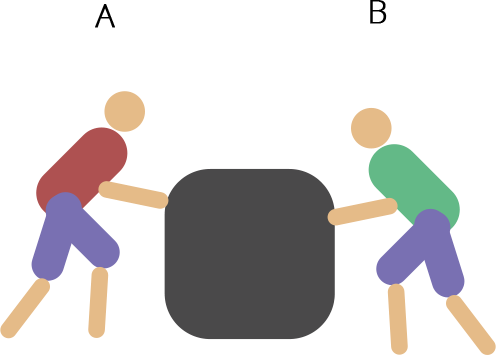(a) Magnitude of force applied by A is greater than that of B.

(b) Magnitude of force applied by A is smaller than that of B.

(c) Net force on the block is towards A.

(d) Magnitude of force applied by A is equal to that of B.

Ans: (a) The force exerted by body A is greater than the force exerted by body B. That’s why the block moves towards the right.

2. In the circuit shown in Fig.11.2, when the circuit is completed, the hammer strikes the gong. Which of the following forces is responsible for the movement of the hammer?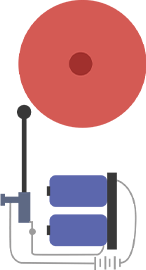(a) gravitational force alone

(b) electrostatic force alone

(c) magnetic force alone

(d) frictional force alone

Ans: (c) The hammer moves due to magnetic force. Electric current flows through the coil which produces an electric current. Due to electric current magnetic force is created.

3. During dry weather, while combing hair, sometimes we experience hair flying apart. The force responsible for this is

(a) force of gravity

(b) electrostatic force

(c) force of friction

(d) magnetic force

Ans: (b) Due to electrostatic force when we comb hair, hair and comb get electrically charged.

4. Fig.11.3 shows a container filled with water. Which of the following statements is correct about the pressure of water?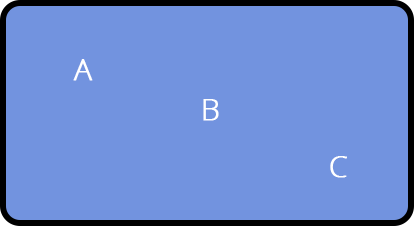(a) Pressure at A> Pressure at B> Pressure at C

(b) Pressure at A=Pressure at B=Pressure at C

(c) Pressure at A< Pressure at B>Pressure at C

(d) Pressure at A< Pressure at B< Pressure at C

Ans: (d) Pressure at A< Pressure at B< Pressure at C. With an increase in depth the pressure of water increases.

5. Two objects repel each other. This repulsion could be due to

(a) frictional force only

(b) electrostatic force only

(c) magnetic force only

(d) either a magnetic or an electrostatic force

Ans: (d) Magnetic and electrostatic forces both possess repel nature.

6. Which one of the following forces is a contact force?

(a) force of gravity

(b) force of friction

(c) magnetic force

(d) electrostatic force

Ans: (b) Force of friction

7. A water tank has four taps fixed at points A, B, C, D as shown in Fig. 11.4. The water will flow out at the same pressure from taps at(a) B and C

(b) A and B

(c) C and D

(d) A and C

Ans: The pressure will remain the same at points B and C because these points are at the same level.

8. A brick is kept in three different ways on a table as shown in Fig. 11.5. The pressure exerted by the brick on the table will be(a) maximum in position A

(b) maximum in position B

(c) maximum in position C

(d) equal in all cases.

Ans: (a) Pressure is inversely proportional to area. That’s why the pressure exerted by brick A will be maximum.

9. A ball of dough is rolled into a flat chapati. Name the force exerted to change the shape of the dough.

Ans: Due to muscular force exerted by the hand the shape of the dough changes.

10. Where do we apply a force while walking?

Ans: We apply pressure on the ground and the reaction force exerted by the ground helps us to move forward.

11. A girl is pushing a box towards the east direction. In which direction should her friend push the box so that it moves faster in the same direction?

Ans: Her friend should push the box towards the east direction to move the box faster in the same direction.

12. In the circuit shown in Fig.11.6, when the key is closed, the compass needle placed in the matchbox deflects. Name the force which causes this deflection.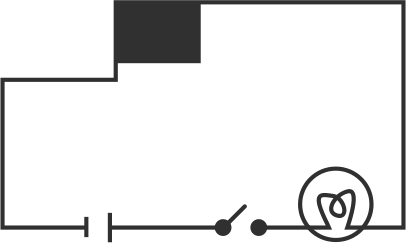Ans: When we close the key, electric current starts flowing in the circuit and due to this a magnetic force is created which deflects the compass’s needle.

13. During dry weather, clothes made of synthetic fibre often stick to the skin. Which type of force is responsible for this phenomenon?

Ans: Due to electrostatic force between the clothes and the body, the clothes of synthetic fibre stick to the body.

14. While sieving grains, small pieces fall down. Which force pulls them down?

Ans: Due to gravitational force the small pieces of sieving grains fall down.

15. Does the force of gravity act on dust particles?

Ans: Yes, the force of gravity also known as a gravitational force acts on the dust particles.

16. A gas-filled balloon moves up. Is the upward force acting on it larger or smaller than the force of gravity?

Ans: The upward force which is helping the balloon to move upward will be greater than the force of gravity.

17. Does the force of gravitation exist between two astronauts in space?

Ans: Yes, gravitational is the universal law that’s why it will also be exerted by the astronauts in space.

18. A chapati maker is a machine that converts balls of dough into chapati’s. What effect of force comes into play in this process?

Ans: This force is called pressure. The pressure exerted by the chapati maker on the dough converts the balls of the dough into chapatis.

19. Fig.11.7 shows a man with a parachute. Name the force which is responsible for his downward motion. Will he come down with the same speed without the parachute?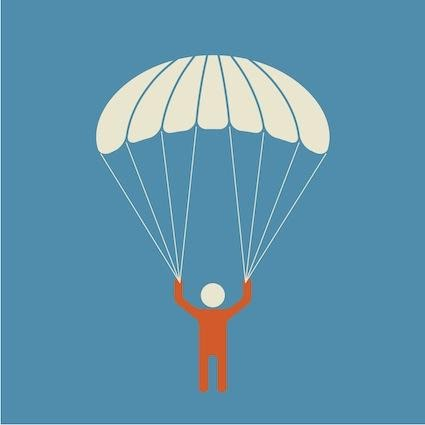Ans: The force responsible for his fall is the force of gravity or the gravitational force. Without a parachute the friction exerted by the man will be less, so he will fall comparatively faster without it.

20. Two persons are applying forces on two opposite sides of a moving cart. The cart still moves with the same speed in the same direction. What do you infer about the magnitudes and direction of the forces applied.

Ans: The magnitude of the forces will cancel each other because these are being exerted in the same direction. With the help of the following figure, we can understand it better.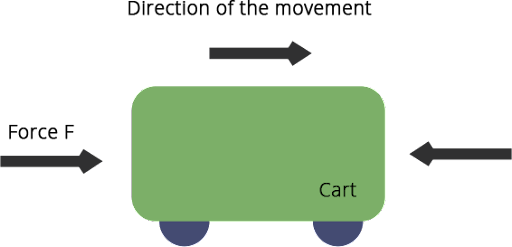21. Two thermocol balls held close to each other move away from each other. When they are released, name the force which might be responsible for this phenomenon. Explain.

Ans: The thermocol balls exert electrostatic force on each other on rubbing. This produces the same charges between them and they start to repel each other.

22. Fruits detached from a tree fall down due to force of gravity. We know that a force arises due to interaction between two objects. Name the objects interacting in this case.

Ans: Earth and the fruit are the interacting objects. Force of gravity is applied by the earth on the fruit that is why it falls down.

23. A man is pushing a cart down a slope. Suddenly the cart starts moving faster and he wants to slow it down. What should he do?

Ans: Following processes can be followed-

(i) He can pull the cart to apply a reaction force on it. It will help to slow down the cart and after some time it may stop.

(ii) By going at the opposite side of the cart he can give the cart the necessary push to slow it down.

In both cases he’ll be applying reaction force on the cart.

24. Fig. 11.8 shows a car sticking to an electromagnet. Name the forces acting on the car? Which one of them is larger?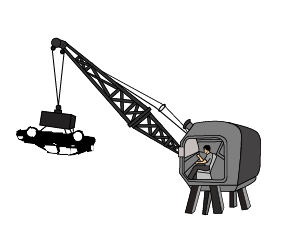Ans: The following forces will be working on the car-

(i) Magnetic Force (upwards)

(ii) Gravitational force or the force of gravity (downwards)

The car is moving upward so we can clearly say that the force acting on the car is electrostatic force.

25. An archer shoots an arrow in the air horizontally. However, after moving some distance, the arrow falls to the ground. Name the initial force that sets the arrow in motion. Explain why the arrow ultimately falls down.

Ans: The force acting on the arrow to move forward is kinetic energy. The gravitational force applies to it vertically and makes it fall on the ground.

26. It is difficult to cut cloth using a pair of scissors with blunt blades. Explain.

Ans: The blunt blade takes more area on the clothes and that’s why the force exerted by the scissors on the clothes becomes less and the cutting of the clothes becomes difficult.

27. Two rods of the same weight and equal length have different thickness. They are held vertically on the surface of sand as shown in Fig.11.9. Which one of them will sink? Why?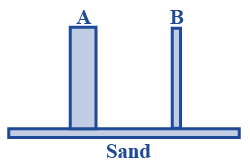Ans: The thinner rod will sink more in the sand. As we know that the pressure exerted by a body is inversely proportional to the area. This helps us to understand that the thinner rod will exert more force on the sand making it sink more.

28. Two women are of the same weight. One wears sandals with pointed heels while the other wears sandals with flat soles. Which one would feel more comfortable while walking on a sandy beach? Give reasons for your answer.

Ans: The woman with flat soles will be more comfortable because the force exerted on the sand by the woman with flat sole will be less. This is because the pressure exerted by the flat sole will be less as compared to the pointed sole and this will help the woman with a flat sole to walk comfortably on the sand.

29. It is much easier to burst an inflated balloon with a needle than by a finger. Explain.

Ans: The needle covers less area as compared to the finger. This helps the needle to exert more force on the balloon to make it blast.

30. Observe the vessels A, B, C and D shown in Fig.11.10 carefully.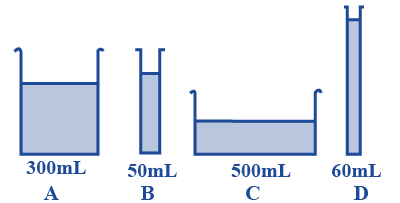The volume of water taken in each vessel is as shown. Arrange them in the order of decreasing pressure at the base of each vessel. Explain.

Ans: The following phenomenon are responsible for the pressure at depth to liquid column-

(i) Density of liquid

(ii) Gravitational acceleration (g)

(iii) Height of liquid

Here Gravitational acceleration and density are the same, so only pressure is the defining criterion so the order of the pressure exerted will be following

PD > PB > PA > PC

### Introduction of chapter 11- Force - NCERT Exemplar Class 8 Science

This chapter helps you to nail your CBSE class 8th exams and also it helps you on other cognitive exams like NTSE and Science Olympiads. Students studying this chapter can develop a strong foundational base in Physic by studying this chapter.

In class 8 Science NCERT Exemplar Solutions Chapter 11-Force, there is an introduction to the topics like force and forces arising from the interaction of objects, change in the state of motion of an object, and contact and non-contact forces. You will also gain knowledge about the concept of pressure and atmospheric pressure in this chapter.

When one or more objects interact with one another then the forces are said to be applied.in physical science, the amount of push or pull acting on an object measures Force.

We add the two forces if they occur in the same direction, and if the two forces occur in the opposite direction then the result of force is given by the difference between the magnitude of these two forces. We measure the strength of force by its magnitude.

### Important Topics Covered Under Chapter 11- FORCE:

• Change in the State of Motion: change in speed of an object or change in direction in which object is moving is referred to as a change in the state of motion. A change in the state of motion will occur if a force is applied to any object at rest or in motion.

• Change in Shape: if force is applied that may also result in a change of shape. For example: if we make chapatis, force is applied which changes its shape.

• Contact Forces-

1. Muscular Forces: the force which is caused by the muscular power of a human or animal on any object is called muscular force. It occurs when the object is in contact with the body that is applying force.

2. Friction: It is applied by the surface in resistance to the motion of an object over it.

• Noncontact Forces-

1. Magnetic Force: It is the forces exerted by two magnets that can be toward or against each other. It can be between the magnet and magnetic object also, this is known as a magnetic force.

2. Electrostatic Force: Force applied by a charged body on a charged or uncharged body is called electrostatic force.

3. Gravitational Force: An attractive force between objects that depends on the mass of two bodies is called Gravitational force.

• Pressure: Force per unit area is called pressure, the atmospheric pressure is the pressure exerted by the atmosphere.

## FAQs on NCERT Exemplar for Class 8 Science Solutions Chapter 11 Force

1. What can a force do?

Force can Bring an object in motion from a state of rest, it can Alter the speed of an object in motion, It can alter the direction of a moving object, It can change the shape of an object.

This chapter is very important and lots of questions are asked out of this chapter. You should prepare this chapter well to score more marks in the exam. Also, you should follow the guidelines to answer the questions correctly and score more marks.

2. What type of questions are asked from Science class8- chapter 11 Force?

There are various types of questions that can be asked from science chapter 11- Force, some of them can be:

• Multiple choice questions- which includes figure-based questions, and choosing the correct or incorrect answers.

• Very short answer type questions-You have to answer in one line

• Figure-based questions-  it is the short answer type questions, in this section, you will answer in the term of force applied due to interaction between two objects.

• 6 long answer questions-  these questions are based on practical situations from our daily life.

3. Why Use NCERT Exemplar Class 8 Science Problems with Solutions Chapter 11 by Vedantu?

Vedantu Experts have given the solutions of NCERT Class 8 Exemplar for Science Chapter 11 according to the CBSE guidelines and format. Vedantu answers will help you get knowledge about how to write an answer in your exam. You can score good marks if you follow the answering pattern given by Vedantu as the solutions provided by Vedantu are the most important part and you can practice them to score good marks in your final exams. You can get one-to-one classes to get a better understanding of the topics.

4. What are the topics covered under Chapter 11 of NCERT Exemplar Solutions for Class 8 Science?

The Exemplar Solutions for Class 8 Science Chapter 11 of NCERT covers the topics like :

• Force – A Push or Pull

• Forces are due to an Interaction

• Exploring Forces

• A Force can Change the State of Motion

• Force can Change the Shape of an Object

• Contact Forces

• Non-Contact Forces

• Pressure

• Pressure Exerted by Liquids and Gasses

• Atmospheric Pressure.

All the topics are described in detail to make the students get a conceptual understanding of the subject. Also, the solutions provide you with a variety of shortcuts that you can opt for to memorize the principles.

5. What are non-contact forces in Chapter 11 of NCERT Exemplar Solutions for Class 8 Science?

The forces that occur without direct contact with the object’s body are known as non-contact forces. Some examples of the non-contact forces are :

• Gravitational force: The strength of gravity is an attractive force that is exerted by the Earth on objects, because of which they fall to the land. The force by which the earth pulls a body is the weight of that body. Students can understand this topic nicely with NCERT Exemplar Solutions prepared by Vedantu’s expert team.

• Electrostatic force: it is the type of force that is acted from a distance this is why it is a non-contact force

• Magnetic force: The force exerted by a magnet on other magnets is called magnetic force.